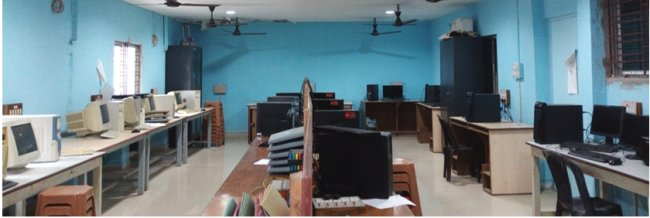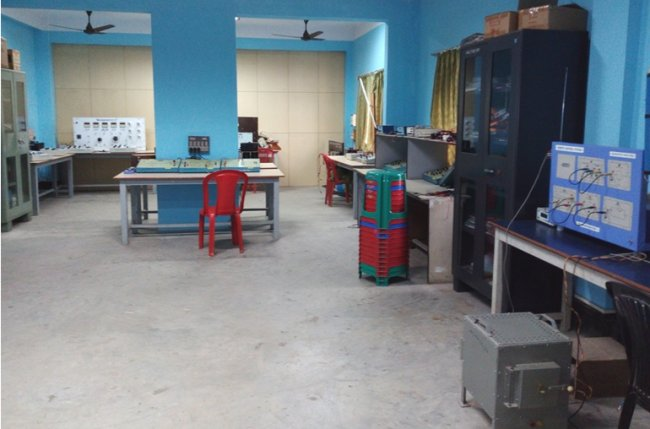::  Laboratories of Applied Electronics & Instrumentation Engineering Department  ::

• LAB 1
• LAB 2
• LAB 3
• LAB 1INDUSTRIAL INSTRUMENTATION LAB(EI-591)
List of Experiments:
1. Calibration of Pressure Gauge using Dead Weight Tester.
2. Study of Thermocouple characteristics and Measurement of Temperature with it.
3. Study of RTD characteristics and Measurement of Temperature with it.
4. Measurements of flow rate and velocity of fluid flow by head type flow meter.
5. Measurements of flow rate and velocity of fluid flow by Variable Area type flow meter.
6. Measurement of level using capacitive type level instrument.
7. Measurement of moisture using moisture analyser.
8. Measurement of viscosity.

SENSORS AND TRANSDUCERS LAB(EI-592)
List of Experiments:
1. Temperature measurement using AD590 IC sensor.
2. Displacement measurement by using a capacitive transducer.
3. Pressure and displacement measurement by using LVDT.
5. Torque measurement Strain gauge transducer.
6. Speed measurement using magnetic proximity sensor.
7. Speed measurement using a Stroboscope.
8. Study of the characteristics of a LDR.

ELECTRONIC INSTRUMENTATION AND MEASUREMENT LAB(EI-692)
List of Experiments:
1. Study of Static Characteristics of a Measuring Instrument
2. Study of Dynamic Characteristics of a Measuring Instrument
3. Acquaintance with basic structure of DMM and measurement of different electrical parameters
4. Realization of Data Acquisition system
5. Wave and spectrum analysis
6. Realization of a V-to-I & I-to-V converter.
7. Statistical analysis of errors in measurement .
8. Study of VCO (Voltage controlled oscillator) & PLL (Phase Locked Loop).

INSTRUMENTATION AND CONTROL DESIGN LAB(EI 892)
List of Experiments:
1) Process Control Loop Design
a. Flow Control
b. Level Control
2) Choose : Controller type, parameters, final control element from specification sheets for a given process functions.
3) Signal to data converter design including coding for different rate variation of signals
4) Corroborate with industry for training / adaptation of the design made in laboratory (software).
5) a. Sensor design and Simulation : specified sensor only
b. Actual sensor design (thick sensors/conventional sensors)
(Collaborate with JU/CGCRI Sensor hub)
6) Controller (digital) design (designing of processor i.e., program) for different process transfer function including dead time (Smith Predictor)
7) Design and fabrication of an instrument like
a. thermal conductivity analyser
b. piezo-electric accelerometer
8) Design of a. specified amplifier
b. counters (high frequency).

• LAB 2CIRCUITS AND NETWORKS LAB [EE(EI)-391]
List of Experiments:
1. Transient response in R-L and R-C Network: Simulation/hardware
2. Transient response in R-L-C Series & Parallel circuits Network: Simulation/hardware
3. Determination of Impedance (Z) and Admittance(Y) parameters of two port network
4. Frequency response of LP and HP filters
5. Frequency response of BP and BR filters
6. Generation of Periodic, Exponential, Sinusoidal, Damped sinusoidal, Step, Impulse, Ramp signals using MATLAB in both discrete and analog form
7. Evaluation of convolution integral, Discrete Fourier transform for periodic & non-periodic signals and simulation of difference equations using MATLAB
8. Representation of poles and zeros in z-plane, determination of partial fraction expansion in z-domain and cascade connection of second order system using MATLAB
9. Determination of Laplace transform and inverse Laplace transformation using MATLAB
10. Spectrum analysis of different signals

MICROPROCESSOR LAB(EI-492)
List of Experiments:
1. a)Familiarization with 8085 trainer kit components.
b) Familiarization with 8085 simulator on PC.
2. a) Study of prewritten programs using basic instruction set (data transfer, Load/Store, Arithmetic, Logical) on the simulator.
b) Assignments based on above
3. PROGRAMMING USING KIT/SIMULATOR FOR
i) Table look up
ii) Copying a block of memory
iii) Shifting a block of memory
iv) Packing and unpacking of BCD numbers
vi) Binary to ASCII conversion
vii) String Matching
viii) Multiplication using Boothï¿½s Algorithm
4. Program using subroutine calls and IN/OUT instructions using 8255 PPI on the trainer kit eg, subroutine for delay, reading switch state & glowing LEDs accordingly, finding out the frequency of a pulse train etc
5. Interfacing any 8-bit Latch (eg, 74LS373) with trainer kit as a peripheral mapped output port with absolute address decoding
6. INTERFACING WITH I/O MODULES:
b) Speed control of mini DC motor using DAC
c) Stepper motor
7. FAMILIARIZATION WITH EPROM PROGRAMMING AND ERASING

CONTROL ENGINEERING LAB [EI 593(EE)]
List of Experiments:
1. Familiarization with MATLAB control system toolbox, MATLAB-SIMULINK toolbox and PSPICE.
2. Study of step response for first and second order system with unity feedback with display on CRT screen and calculation of parameters for different system designs.
3. Simulation of impulse response for types 0, 1 and 2 with unity feedback using MATLAB and PSPICE.
4. Determination of root-locus, Bode plot, Nyquist plot using MATLAB toolbox for a given second order transfer function and listing of the specifications.
5. Determine the effect of P, I, D actions on first order simulated process and obtaining the system transfer functions from Bode plot.

List of Experiments:
1. Familiarization with 8086/88 trainer kit components.
2. a) Study of prewritten programs on trainer kit using the basic instruction set (data transfer, Load/Store, Arithmetic, Logical)
b) Assignments based on above.
3. a) Familiarization with 8086/88 simulator on PC.
b) Study of prewritten programs using basic instruction set (data transfer, Load/ Store, Arithmetic, Logical) on the simulator.
c) Assignments based on above
4. PROGRAMMING USING KIT/SIMULATOR FOR
Table look up
i) Copying a block of memory
ii) Shifting a block of memory
iii) Packing and unpacking of BCD numbers
v) Binary to ASCII conversion
vi) String Matching
vii) Sorting etc.
5. Program using subroutine calls and IN/OUT instructions using 8255 PPI on the trainer kit e.g., subroutine for delay,
reading switch state & glowing LEDs accordingly, finding out the frequency of a pulse train etc
6. INTERFACING WITH I/O MODULES:
ii) Speed control of mini DC motor using DAC
iii) Temperature sensor and display temperature
iv) Relay
v) Keyboard through 8279 and 8255A
vi) Multi-digit Display with multiplexing through 8255A & 8279
vii) Stepper motor
7. Study of 8051 Micro controller kit and writing programs for the following tasks using the kit.
a) Table look up.
b) Basic arithmetic and logical operations.
c) Interfacing of Keyboard and stepper motor.

POWER ELECTRONICS LAB[EI 891A(EE)]
List of Experiments:
1. Study of V-I Characteristics of an SCR and a TRIAC.
2. Study of Diode-Resistance, Diode-Resistance-Capacitance, Resistance-Capacitance and UJT Triggering Circuits for SCR.
3. Study of the operation of a single phase fully controlled bridge converter supplying R-L load and free wheeling diode, including generation of triggering pulses for the devices for both continuous and discontinuous modes of conduction.
4. Study of a self commutation circuit for commutating an SCR operating on a DC supply.
5. Simulation of DC to DC step down chopper.
6. Simulation of PWM bridge inverter using MOSFET/IGBT with R/R-L load.
7. Simulation of Single phase AC regulator.
8. Study of a control circuit for a stepper motor and its operation./ Study of a single quadrant chopper controlled PM dc motor.

• LAB 3ELECTRICAL MEASUREMENT & INSTRUMENTATION LAB(EI-491)
List of Experiments:
1. Instrument workshop- observe the construction of PMMC, Dynamometer, Electro thermal and Rectifier type instrument, Oscilloscope and digital multimeter.
2. Calibrate moving iron and electrodynamometer type ammeter/voltmeter by potentiometer
3. Calibrate dynamometer type Wattmeter by potentiometer
4. Calibrate A.C. energy meter.
5. Measure the resistivity of material using Kelvin Double Bridge
6. Measurement of Power using Instrument transformer
7. Measurement of Inductance by Anderson Bridge
8. Measurement of Capacitance by De Sauty Bridge.

TELEMETRY AND REMOTE CONTROL LAB(EI-791)
List of Experiments:
1. Study of voltage telemetry system using a process variable transducer.
2. Study of 4-20 mA current telemetry system: 2 wire and 3 wire systems.
3. Study of a frequency telemetry system using a VCO and a PSD.
4. Study of a FDM and Demultiplexing system using wire transmission for 2 to 4 channels.
5. Study of a PCM system.
6. Study of a Bio - Telemetry System.
7. Study of a (wireless) remote control system.
8. Study of Computerized control wireless telemetry system.